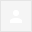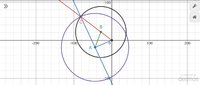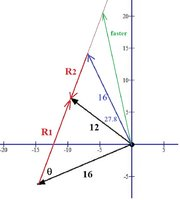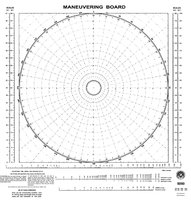# Navigation: fast ship course and ETA to intercept slow ship

#### perlboy

##### New member
Two ships leave port at 9 a.m. One travels at a bearing of N 53 degrees W at 12 miles per hour, and the other travels at a bearing of S 67 degrees W at 16 miles per hour. 1) Approximately how far apart are the ships at noon that day; and 2) what bearing and ETA should the faster ship set to intercept the slower if the slower continues on its original course and speed?

The first part is easy to solve with the Law of Cosines. First, determine the vector magnitudes: 3 x 12 = 36 and 3 x 16 = 48. Then determine the enclosing angle: (180 - 143 = 37) + (270 - 67 - 180 = 23) = 60 degrees. Then, a2 = 362 + 482 - 2(36){48)cos 60 = 1872. Therefore, the distance between the ships is the square root of 1872: 43.27 miles.

The next part is not so easy. I can determine the X,Y cordinates of the two ships by converting from polar to Cartesian coordinates; slower (-28.75, 21.67), faster (-44.18, -18.76), but I am at a loss how to calculate the bearing and magnitude of the faster vector and the magnitude of the slower vector. Perhaps there isn't enough information to set up an appropriate equation, but I doubt it. Naval navigators must be able to quickly solve this problem. Any suggestions how to approach part 2 will be greatly appreciated.

#### lev888

##### Senior Member
Two ships leave port at 9 a.m. One travels at a bearing of N 53 degrees W at 12 miles per hour, and the other travels at a bearing of S 67 degrees W at 16 miles per hour. 1) Approximately how far apart are the ships at noon that day; and 2) what bearing and ETA should the faster ship set to intercept the slower if the slower continues on its original course and speed?

The first part is easy to solve with the Law of Cosines. First, determine the vector magnitudes: 3 x 12 = 36 and 3 x 16 = 48. Then determine the enclosing angle: (180 - 143 = 37) + (270 - 67 - 180 = 23) = 60 degrees. Then, a2 = 362 + 482 - 2(36){48)cos 60 = 1872. Therefore, the distance between the ships is the square root of 1872: 43.27 miles.

The next part is not so easy. I can determine the X,Y cordinates of the two ships by converting from polar to Cartesian coordinates; slower (-28.75, 21.67), faster (-44.18, -18.76), but I am at a loss how to calculate the bearing and magnitude of the faster vector and the magnitude of the slower vector. Perhaps there isn't enough information to set up an appropriate equation, but I doubt it. Naval navigators must be able to quickly solve this problem. Any suggestions how to approach part 2 will be greatly appreciated.
Not enough info for a unique solution. Maybe you are supposed to provide a solution. Try to find one that results in fastest interception.

#### jonah2.0

##### Full Member
Two ships leave port at 9 a.m. One travels at a bearing of N 53 degrees W at 12 miles per hour, and the other travels at a bearing of S 67 degrees W at 16 miles per hour. 1) Approximately how far apart are the ships at noon that day; ...

The first part is easy to solve with the Law of Cosines. First, determine the vector magnitudes: 3 x 12 = 36 and 3 x 16 = 48. Then determine the enclosing angle: (180 - 143 = 37) + (270 - 67 - 180 = 23) = 60 degrees. Then, a2 = 362 + 482 - 2(36){48)cos 60 = 1872. Therefore, the distance between the ships is the square root of 1872: 43.27 miles.
Roger that.
and 2) what bearing and ETA should the faster ship set to intercept the slower if the slower continues on its original course and speed?
We're gonna need an opinion from retired navy officer skeeter on this one.

Last edited:

#### Dr.Peterson

##### Elite Member
Two ships leave port at 9 a.m. One travels at a bearing of N 53 degrees W at 12 miles per hour, and the other travels at a bearing of S 67 degrees W at 16 miles per hour. 1) Approximately how far apart are the ships at noon that day; and 2) what bearing and ETA should the faster ship set to intercept the slower if the slower continues on its original course and speed?

The first part is easy to solve with the Law of Cosines. First, determine the vector magnitudes: 3 x 12 = 36 and 3 x 16 = 48. Then determine the enclosing angle: (180 - 143 = 37) + (270 - 67 - 180 = 23) = 60 degrees. Then, a2 = 362 + 482 - 2(36){48)cos 60 = 1872. Therefore, the distance between the ships is the square root of 1872: 43.27 miles.

The next part is not so easy. I can determine the X,Y cordinates of the two ships by converting from polar to Cartesian coordinates; slower (-28.75, 21.67), faster (-44.18, -18.76), but I am at a loss how to calculate the bearing and magnitude of the faster vector and the magnitude of the slower vector. Perhaps there isn't enough information to set up an appropriate equation, but I doubt it. Naval navigators must be able to quickly solve this problem. Any suggestions how to approach part 2 will be greatly appreciated.
Here's how I solved it: If the fast ship is now at A and the slow one at B, they will intercept at C on OB extended. We need AC/BC = 16/12 since they continue at the same speeds. Use the law of sines to find the required angle CAB. The rest should follow.

•jonah2.0

#### jonah2.0

##### Full Member
Beer soaked ramblings follow.
2) what bearing and ETA should the faster ship set to intercept the slower if the slower continues on its original course and speed?
Playing with Desmos, I slowly adjusted the radius of circles centered at P_1 and P_2 till they "seemed" to intersect with the line y=tan(pi/2+(53*pi)/180)*x at (-82.145, 61.901)

Last edited:

#### perlboy

##### New member7:38 PM (6 minutes ago)

The approach to solving this problem turned out to be very different than I expected it to be. My initial attempts were based on what Dr. Peterson suggested; namely, that the ratio of two legs of one of the angles was 16 ÷ 12. Unfortunately, what is needed here is 16x ÷ 12x where x represents time. Without solving for x it is impossible to determine ETA.

I have a Sharp EL W516 scientific calculator, which has an equation solver function that uses Newton's method to solve for roots. I used the following equation to solve for two of the angles which determine the vector magnitudes needed for the fast ship to intercept the slow ship.

[ 43.3 sin (74 - x) ÷ 12 sin (x) ] - [ 43.3 sin (106) ÷ 16 sin (x) ]

Using Dr. Peterson's notation, the angle at B determined with the Law of Sines is 74 degrees, rounded for simplicity to whole numbers. Its supplement is 106, therefore, the two remaining angles relevant to part 2 must add to 74. I chose 40 as a start "guess" and dx = 0.01. The solution to the above equation is:

x = 27.867°, and ∴ 74 - x = 46.133°

This leads directly with Law of Sines to distance traveled in miles:

12x = 66.786
16x = 89.047

Dividing by 12 and 16 respectively yields ETA = 5.566 ≈ 5 hrs 34 mins.

∴ The course set by the fast ship would be 270 - 67 - 46.133 ≈ 157°.

Notice that 89.047 ÷ 66.786 ≈ 16 ÷ 12 or 1 ⅓

Essentially, using the equation solver is equivalent to how most Calculus texts introduce finding a limit, by choosing appropriate ϵ values and then determining a 𝛅 until the function converges at its limit, if it exists.

#### Dr.Peterson

##### Elite Member
The approach to solving this problem turned out to be very different than I expected it to be. My initial attempts were based on what Dr. Peterson suggested; namely, that the ratio of two legs of one of the angles was 16 ÷ 12. Unfortunately, what is needed here is 16x ÷ 12x where x represents time. Without solving for x it is impossible to determine ETA.
You don't need to solve for x to find the value of (16x)/(12x)! That's what ratios are all about!

And the law of sines is all about ratios.

#### perlboy

##### New member
Yes, I know the Law of Sines is about ratios. Please, show me how you would determine ETA by your method? Solving for ETA is part of the original problem. That is exactly what I am looking for. I showed you mine, in detail. Please show me yours. As we say in perl culture: TMTOWTDI.

#### jonah2.0

##### Full Member
Beer soaked ramblings follow.7:38 PM (6 minutes ago)

The approach to solving this problem turned out to be very different than I expected it to be. My initial attempts were based on what Dr. Peterson suggested; namely, that the ratio of two legs of one of the angles was 16 ÷ 12. Unfortunately, what is needed here is 16x ÷ 12x where x represents time. Without solving for x it is impossible to determine ETA.

I have a Sharp EL W516 scientific calculator, which has an equation solver function that uses Newton's method to solve for roots. I used the following equation to solve for two of the angles which determine the vector magnitudes needed for the fast ship to intercept the slow ship.

[ 43.3 sin (74 - x) ÷ 12 sin (x) ] - [ 43.3 sin (106) ÷ 16 sin (x) ]

Using Dr. Peterson's notation, the angle at B determined with the Law of Sines is 74 degrees, rounded for simplicity to whole numbers. Its supplement is 106, therefore, the two remaining angles relevant to part 2 must add to 74. I chose 40 as a start "guess" and dx = 0.01. The solution to the above equation is:

x = 27.867°, and ∴ 74 - x = 46.133°

This leads directly with Law of Sines to distance traveled in miles:

12x = 66.786
16x = 89.047

Dividing by 12 and 16 respectively yields ETA = 5.566 ≈ 5 hrs 34 mins.

∴ The course set by the fast ship would be 270 - 67 - 46.133 ≈ 157°.

Notice that 89.047 ÷ 66.786 ≈ 16 ÷ 12 or 1 ⅓

Essentially, using the equation solver is equivalent to how most Calculus texts introduce finding a limit, by choosing appropriate ϵ values and then determining a 𝛅 until the function converges at its limit, if it exists.

Using Dr.Peterson's reference points,AB=sqrt1872=12sqrt13approx43.2666 miles
(sinangleABO)/48=(sin60°)/(12sqrt13)impliesangleABO=sin^(-1)((48sin60°)/(12sqrt13))approx73.8979°
Thus, in triangle ABC, angleB=180°-angleABOapprox106.1021°
For the rest of triangle ABC, we have
(sinangleA)/12=(sinangleB)/16impliesangleA=sin^(-1)(12*(sinangleB)/16)approx46.1021°
angleC=180°-angleA-angleBapprox27.7958°
We then have
(AC)/sinB=(AB)/sinC\LeftrightarrowAC=sinB*(AB)/sinCapprox89.1429 miles

and

(BC)/sinA=(AB)/sinC\LeftrightarrowBC=sinA*(AB)/sinCapprox66.8571 miles

In accordance with the bearing used by the problem statement,
the faster ship must then set a bearing of N 25.2042° degrees W from point A where

tan^(-1)((48sin(270°-67°)-(36+BC)sin(90°+53°))/(48cos(270°-67°)-(36+BC)cos(90°+53°)))+180°-90°approx25.2042°

For the ETA, we have (AC)/16=(BC)/12approx5.5714 hours

This of course is under the unrealistic scenario where the faster ship can turn at an angle of 46.1021° without losing its momentum like a light ray "bouncing" off a mirror. And there's the added question of what it means to intercept another ship (It must be a wartime situation of some sort). Is the faster ship intending to ram the slower ship? Maybe it will just fire its guns upon sighting the slower ship. But then detection by radars and plain eyesight. Maybe there's a peaceful situation application that I can't think of right now. Need more beer.

#### lev888

##### Senior Member
Have to ask: if it's obvious that the faster ship maintains its speed, why does the problem state that the slower ship "continues on its original course and speed"?

#### Dr.Peterson

##### Elite Member
In case it isn't clear, the following line reflects my suggestion:
For the rest of triangle ABC, we have
(sinangleA)/12=(sinangleB)/16impliesangleA=sin^(-1)(12*(sinangleB)/16)approx46.1021°
This is equivalent to
(sinangleA)/(sinangleB)=12/16
This of course is under the unrealistic scenario where the faster ship can turn at an angle of 46.1021° without losing its momentum like a light ray "bouncing" off a mirror. And there's the added question of what it means to intercept another ship (It must be a wartime situation of some sort). Is the faster ship intending to ram the slower ship? Maybe it will just fire its guns upon sighting the slower ship. But then detection by radars and plain eyesight. Maybe there's a peaceful situation application that I can't think of right now. Need more beer.
Clearly, if you are captain A, you suddenly realized that all your beer had been left on ship B, so you had to go to C to retrieve it. And that increases your skill in making fast turns.

Have to ask: if it's obvious that the faster ship maintains its speed, why does the problem state that the slower ship "continues on its original course and speed"?
I'm just following the word-problem convention of making whatever assumptions I can in order to make the problem solvable. Presumably each ship was going at its maximum speed, and continues doing so.

As the navigator in real life, I would of course ask, "At what speed, sir?"

•jonah2.0

#### perlboy

##### New member
Cool! This discussion is exactly why I posted this problem here, to hopefully get an interesting dialogue going that would shed light on the beauty of trigonometry. I was not looking for someone to help me solve a homework problem. I came across a problem that interested me, i.e., the part 1 scenario. I added the part 2 question, because I wanted to see whether I could solve it, and was stumped enough to post it here. I then tried my calculator's built in function, somewhat like using desmos, or possibly even Ptolemy, and got an answer, but I knew there must be other approaches to a solution. Bravo and thanks to all.

Yes, assumptions are, I can play that game too, that both vessels are already traveling at maximum speed, that faster vessel does not wish to alert slower captain that he will be under attack, thus slower proceeds fat, dumb and happy, and earliest intercept is desired. Turning 46 degrees to starboard takes less than a minute, and I used ≈ to imply rounding. Back before homing torpedoes, that was a problem solved by a submarine's attack computer, to determine angle on the bow and runtime. We got the faster vessel running hot, straight and true.

#### perlboy

##### New member
My bad! I got the new ship's heading very wrong. If I had offered that course change the ship would have missed the target by many miles. I would have been banished from the bridge and put in hack. Thank you jonah2.0 for catching my mistake. But jeze Louise, that is one complex arctan calc. It proves you are a virtuoso trig aficionado, way more than me. I'm just a lowly perl hacker. But why not, to save a bunch of time...

180 - (∠CAB + ∠BAO) = 87.7958

then

180 - (67 + 87.7958) = 25.2042, which I concur is N 25.2042° W.

So the order should have been "come right to new course 115° 12' 15.1" true."Just 115 would be cool and then I would allow for set and drift in the dead reckoning plot.

BTW: ∠CAB = ∠BAO, but only because the faster vessel was not permitted to alter its speed.

•jonah2.0

#### perlboy

##### New member
So the order should have been "come right to new course 334° 47' 44.9" true."Just 335 would be cool and then I would allow for set and drift in the dead reckoning plot.

•jonah2.0

#### Dr.Peterson

##### Elite Member
BTW: ∠CAB = ∠BAO, but only because the faster vessel was not permitted to alter its speed.
Are you saying you knew all along that ∠CAB = ∠BAO, or that you just noticed it in the answer?

As soon as I saw that fact stated, I realized that it is "obvious" -- in fact, it is implied by the very ratio I pointed out at the start. The angle bisector theorem says that because OA:OB = AC:BC, therefore AB is the bisector of angle OAC. So once we'd solved triangle OAB, C was all but located.

I hadn't considered using geometric theorems because the problem is a typical trig problem, or so it seemed.

•jonah2.0

#### jonah2.0

##### Full Member
Beer soaked ramblings follow.
Clearly, if you are captain A, you suddenly realized that all your beer had been left on ship B, so you had to go to C to retrieve it. And that increases your skill in making fast turns.
That is one fascinating conjecture.

#### skeeter

##### Elite Member
In actual practice, ship navigation/collision avoidance used to be done exclusively on a sheet of maneuvering board paper (see attached) with pencil, dividers, and parallel rulers. Distances were measured with dividers and speed/time calculations were done using nomograms on the sheet. From what I've heard, technology has taken over, but manual calculations are still practiced in case the technology fails.
The basic concept behind the maneuvering board is relative motion as a vector. "Own" ship is always at the center, and contacts are plotted over time to view the relative motion and calculate the other ships' course & speed.

In solving the posted problem (see attached graph), the relative motion vector has speed, $$\displaystyle R_1 = 4\sqrt{13}$$ knots, and shows the initial direction of the slower ship relative to the faster. Over 3 hours, the slower ship is a distance of $$\displaystyle 12\sqrt{13}$$ miles from the faster. To intercept, all that is needed is to reverse the direction of $$\displaystyle R_1$$, yielding $$\displaystyle R_2 \approx 7.8$$ knots, which will take about 5 hrs + 34 min to intercept. Note the faster ship's change in course while maintaining the same speed. The direction can be read right off the maneuvering board's polar-type grid. If a sooner intercept is required, the faster ship obviously needs to increase speed (green vector) and have it's motion vector touch the relative motion vector's path.•jonah2.0

#### perlboy

##### New member
Are you saying you knew all along that ∠CAB = ∠BAO, or that you just noticed it in the answer?
I should have, but I didn't. I did observe that ∠CAB = ∠BAO but did not initially appreciate its significance. I calculated ∠ABO and ∠BAO after first determining the distance between the two points with Law of Cosines. Then, I determined ∠ABC as the supplement of ∠ABO and that's where I got stuck. I did not accept your suggestion that all that was needed to solve the two remaining angles was the ratio 12:16. I convinced myself that I could place ∠ACB anywhere along the line BC, but it would not align with AC without taking time into consideration. In other words, I missed the significance of proportionality.

To solve the time element I resorted to my calculator's solve function to find its limit, and therein noted ∠CAB = ∠BAO. The penny still didn't drop, so I had to resort to a thought experiment. I drew two vectors at an arbitrary angle, as 12/0 and 16/ ≈ 30 and connected their endpoints. I then multiplied both magnitudes by 4 and again drew a line connecting their endpoints. I noted these two lines were parallel, and then the light bulb lit up, and I knew you were right, that the problem could be solved by only knowing the ratios 12:16 and 36:48, but again, as long as both ships maintained original course and speed. I also completely solved two other similar problems with different speeds and interior angles with my calculator and again noted the equality ∠CAB = ∠BAO, but again, without resorting to, or being aware of the theorem.

So, a navigator well versed in trig and geometry could answer this and another interesting question almost instantly, or at least, it would appear to be such to observers on the bridge, and get himself out of hack. You said, "At what speed, sir?" The first answer would be, "if we maintain current speed, the course change is 335 true and the ETA is 5 hrs 34 mins. Now if we increase speed to say 20 knots (knots is correct but miles was used in the original statement of the problem,) then the course is 328 true with ETA 3 hrs 20 mins."

sin-1 ( (12 sin 106) / 20) = 35.222°
360 - (180 - (67 + 46.1021 + 35.222)) ≈ 328° true.

ETA is:

[ 43.3 sin (106) ÷ 20 sin (73.8979-35.222) ] = 3.330 = 3 hrs 20 mins.

The ratio would now be 12:20 and changing one number, 16 to 20 would quickly result in the value for ∠CAB, namely 35.222°. The calculator is no longer needed, but could still be used as fail-safe, to verify the math.

I intuited there was a principle involved here, as my first expression of new course to target was 180 - (67 + 2(46.1021)) = 25.2042°, but then I changed that to 180 - (67 + 46.1021 + 46.1021) = 25.2042° to make it more general, in anticipation that a speed change might be requested. You gave this notion a name, angle bisector theorem, which I may have studied many years ago in HS geometry (I minored in math at U of I Chicago but that was class of '71, eons ago), but that was so long ago I'd forgotten it. Thanks for naming it, since that saved me a google search, out of native curiosity.

•jonah2.0